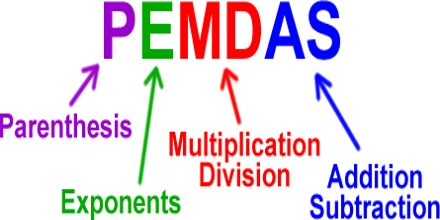Mathematic

# Order of OperationsThe aim of this lecture is to present on Order of Operations. Order of Operations are a standard way to simplify mathematical expressions and equations. Purpose of this term: Avoids Confusion and Gives Consistency. Order of operations are a set of rules that mathematicians have agreed to follow to avoid mass confusion when simplifying mathematical expressions or equations. Without these simple, but important rules, learning mathematics would be maddening.